# Modeling bumblebee colony growth with a switch point

library(bumbl)
library(car)
#> Loading required package: carData

## Introduction to the bumbl() function

Bumblebee colony growth is characterized by an initial exponential growth period when workers are being produced, followed by a switch to production of reproductive individuals (gynes and drones). Reproductive individuals leave the nest, resulting in a decline in colony size. The initial growth rate, the rate or decline, and the time to switching to reproduction may vary in response to environmental conditions (Crone and Williams 2016).

The bumbl() function fits a model, described below and in Crone & Williams (2016), that finds a growth rate ($$\lambda$$), a decline rate ($$\delta$$) , and a switchpoint ($$\tau$$). When supplied with a dataset with multiple colonies, a model is fit separately for each colony and these three parameters (as well as an estimated starting colony size and maximum colony size) are returned. In this example, we’ll use the built-in bombus dataset. For more information on this dataset see the help file with ?bombus.

Before the switch point, growth (colony weight, $$W_t$$) is defined as:

$W_t = \lambda^tW_0$ After the switchpoint ($$t > \tau$$), it’s defined as:

$W_t = \lambda^{\tau}W_{0}\delta^{t-\tau}$

Where $$\delta$$ is a rate of decline. Therefore:

$W_t = \begin{cases} \lambda^tW_0 & t\leq\tau \\ \lambda^{\tau}W_{0}\delta^{t-\tau} & t > \tau \end{cases}$

After log-linearizing this model, it it looks like this:

For $$t \leq \tau$$:

$\ln(W_t) = \ln(W_0) + t\ln(\lambda)$

For $$t>\tau$$

$\ln(W_t) = \ln(W_0) + \tau\ln(\lambda) + (t-\tau)\ln(\delta)$

bumbl() works by treating this as a generalized linear model with a log-link after creating a new variable, .post which is 0 before $$\tau$$ and $$t-\tau$$ after $$\tau$$. Then the value of $$\tau$$ that maximizes likelihood is found and used to fit a final model.

$ln(W_t) = \beta_0 + \beta_1t + \beta_2 \textrm{ .post}$

To clarify, in the above equation:

• $$\beta_0 = \ln(W_0)$$
• $$\beta_1 = \ln(\lambda)$$
• $$\beta_2 = \ln(\delta-\lambda) = \frac{\ln(\delta)}{\ln(\lambda)}$$

## Modeling Bombus colony growth

head(bombus)
#> # A tibble: 6 × 10
#>   site  colony  wild habitat date        week  mass d.mass floral_resources
#>   <fct> <fct>  <dbl> <fct>   <date>     <int> <dbl>  <dbl>            <dbl>
#> 1 PUT2  9       0.98 W       2003-04-03     0 1910.    0.1            27.8
#> 2 PUT2  9       0.98 W       2003-04-09     1 1940    30.6            27.8
#> 3 PUT2  9       0.98 W       2003-04-15     2 1938    28.6            27.8
#> 4 PUT2  9       0.98 W       2003-04-22     3 1976.   67.1            27.8
#> 5 PUT2  9       0.98 W       2003-05-01     4 2010.  101.              7.96
#> 6 PUT2  9       0.98 W       2003-05-07     5 2143   234.              7.96
#> # … with 1 more variable: cum_floral <dbl>

The bumbl() function expects a tidy dataset with a column for some measure of time, and a column for some measure of colony size at minimum. A formula is required, which at minimum should have colony size on the left-hand side, and time on the right-hand side.

out <- bumbl(bombus, colonyID = colony, t = week, formula = d.mass ~ week)
#> Warning: Search for optimal switchpoint did not converge for colonyID '32'. Omitting from results.
#> Warning: Search for optimal switchpoint did not converge for colonyID '71'. Omitting from results.
#> # A tibble: 6 × 7
#>   colony converged   tau logN0 logLam  decay logNmax
#>   <chr>  <lgl>     <dbl> <dbl>  <dbl>  <dbl>   <dbl>
#> 1 104    TRUE       6.42  3.44  0.380 -0.541    5.78
#> 2 14     TRUE       6.40  4.04  0.295 -0.458    5.83
#> 3 17     TRUE       6.36  3.39  0.407 -0.662    5.83
#> 4 20     TRUE       7.27  2.79  0.194 -0.345    4.14
#> 5 22     TRUE       6.92  2.65  0.280 -0.439    4.57
#> 6 24     TRUE       6.23  4.06  0.167 -0.391    5.06

For each colony, we get the maximum likelihood estimate for $$\tau$$ (tau), the estimated initial colony weight ($$\ln({W_0})$$, logN0) on a log scale, the average colony growth rate ($$\ln(\lambda)$$, logLam) on a log scale, the rate of decline after $$\tau$$ ($$ln(\delta - \lambda)$$, decay), and the estimated maximum weight of each colony, on a log scale (logNmax).

The supplied formula can also include covariates for colony growth. The model coefficients for any additional covariates are included in the output of bumbl(). Here, I’ve included cumulative floral resources as a covariate. Accounting for some covariates, such as time of day, might give better estimates of the switchpoint or growth and decay rates.

out2 <- bumbl(bombus, colonyID = colony, t = week,
formula = d.mass ~ week + cum_floral)
#> Warning: Search for optimal switchpoint did not converge for colonyID '20'. Omitting from results.
#> Warning: Search for optimal switchpoint did not converge for colonyID '67'. Omitting from results.
#> Warning: Search for optimal switchpoint did not converge for colonyID '86'. Omitting from results.
#> # A tibble: 6 × 8
#>   colony converged   tau logN0 logLam  decay logNmax beta_cum_floral
#>   <chr>  <lgl>     <dbl> <dbl>  <dbl>  <dbl>   <dbl>           <dbl>
#> 1 104    TRUE       5.56  3.12  0.361 -0.633    5.73         0.0142
#> 2 14     TRUE       5.57 -4.63 -0.381 -0.534    5.78         0.154
#> 3 17     TRUE       8.47  3.99  0.567 -0.545    5.82        -0.0267
#> 4 22     TRUE       7.11  2.87  0.409 -0.491    4.59        -0.00960
#> 5 24     TRUE       6.40  4.04  0.185 -0.386    5.04        -0.00174
#> 6 26     TRUE       7.28  3.34 -0.158 -0.111    5.49         0.0223

You may also use count data, such as number of workers entering or exiting a hive, with either a Poisson or negative binomial GLM by supplying the family argument.

## Checking Results

As is the case with any GLM, some model diagnostics should be performed before interpreting the results. One way to check that results are sensible, is to plot them. The plot() method for data frames produced by bumbl() (plot.bumbldf()) plots each colony’s observed and estimated growth trajectory as a separate plot. If you only want to display certain results, you can supply a vector of indices or colony ID’s to the colony argument.

plot(out, colony = c("17", "104", "20", "24"))
#> Creating plots for 4 colonies...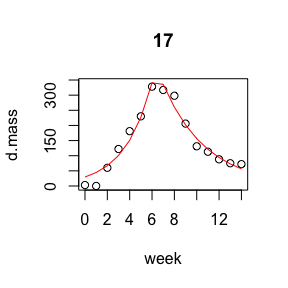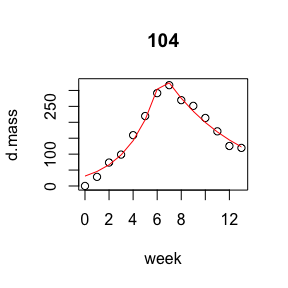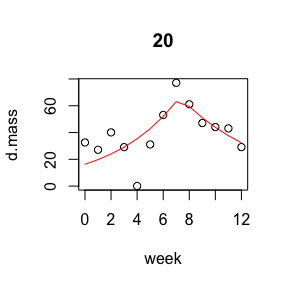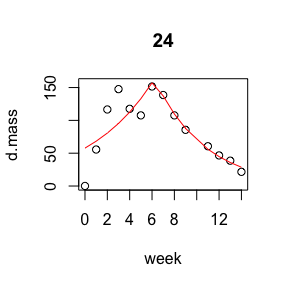Observed values for colony 20 don’t follow a neat growth and decline shape, and the colony mass was consistently very low. Because of this, we might not trust the value for tau as representing a true switch from workers to reproductives.

There is also an autoplot() method that can be used if you have the ggplot2 package installed. It returns a ggplot object which can be modified.

library(ggplot2)
p <- autoplot(out, colony = c("17", "104", "20", "24"))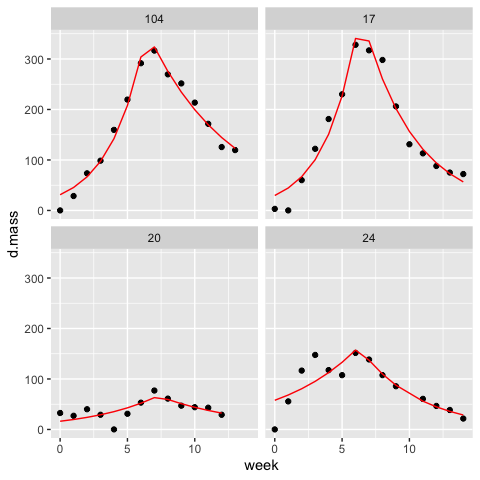p + theme_bw()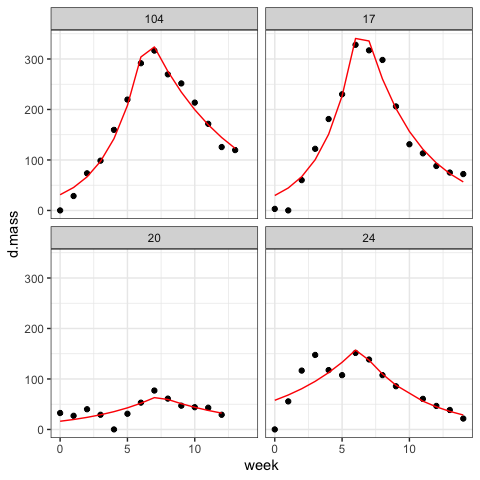If you’d rather get these data and produce your own plots, run bumbl() with augment = TRUE to get fitted values to plot.

Other model diagnostics (plots or statistics) can be obtained from the GLMs fit to each colony. Running bumbl() with keep.model = TRUE produces an output with a list-column containing the GLMs.

out3 <- bumbl(bombus, colonyID = colony, t = week, formula = d.mass ~ week, keep.model = TRUE)
#> Warning: Search for optimal switchpoint did not converge for colonyID '32'. Omitting from results.
#> Warning: Search for optimal switchpoint did not converge for colonyID '71'. Omitting from results.
#> # A tibble: 6 × 8
#>   colony model  converged   tau logN0 logLam  decay logNmax
#>   <chr>  <list> <lgl>     <dbl> <dbl>  <dbl>  <dbl>   <dbl>
#> 1 104    <glm>  TRUE       6.42  3.44  0.380 -0.541    5.78
#> 2 14     <glm>  TRUE       6.40  4.04  0.295 -0.458    5.83
#> 3 17     <glm>  TRUE       6.36  3.39  0.407 -0.662    5.83
#> 4 20     <glm>  TRUE       7.27  2.79  0.194 -0.345    4.14
#> 5 22     <glm>  TRUE       6.92  2.65  0.280 -0.439    4.57
#> 6 24     <glm>  TRUE       6.23  4.06  0.167 -0.391    5.06

Say, for example, you want to compute an R2 value using the rsq package.

library(dplyr)
#>
#> Attaching package: 'dplyr'
#> The following object is masked from 'package:car':
#>
#>     recode
#> The following objects are masked from 'package:stats':
#>
#>     filter, lag
#> The following objects are masked from 'package:base':
#>
#>     intersect, setdiff, setequal, union
library(purrr)
#>
#> Attaching package: 'purrr'
#> The following object is masked from 'package:car':
#>
#>     some
library(rsq)
#for a single colony
# m <- out3\$model[]
# rsq(m)

#for all colonies
out3.1 <-
out3 %>%
mutate(r2 = purrr::map_dbl(model, rsq), .after = model)

out3.1 %>% filter(colony %in% c("104", "17", "20", "24"))
#> # A tibble: 4 × 9
#>   colony model     r2 converged   tau logN0 logLam  decay logNmax
#>   <chr>  <list> <dbl> <lgl>     <dbl> <dbl>  <dbl>  <dbl>   <dbl>
#> 1 104    <glm>  0.977 TRUE       6.42  3.44  0.380 -0.541    5.78
#> 2 17     <glm>  0.956 TRUE       6.36  3.39  0.407 -0.662    5.83
#> 3 20     <glm>  0.473 TRUE       7.27  2.79  0.194 -0.345    4.14
#> 4 24     <glm>  0.729 TRUE       6.23  4.06  0.167 -0.391    5.06

We can see that colony 20 has a much lower R2 value than colonies 104, 17, and 24 which matches our expectations from plotting the observed and fitted values.

See vignette("nest", package = "tidyr") for more about working with list-columns containing models.